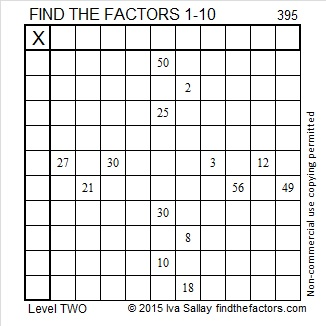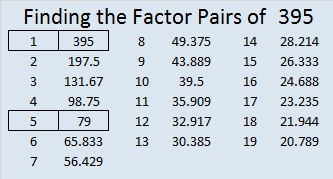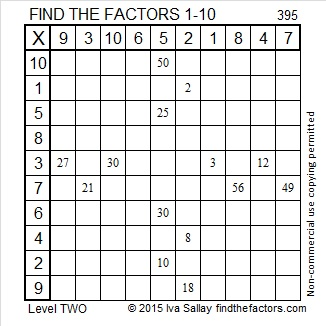# 395 and Level 2

395 ends with a five so it is a composite number that is divisible by 5.Print the puzzles or type the factors on this excel file: 10 Factors 2015-02-16

• 395 is a composite number.
• Prime factorization: 395 = 5 x 79
• The exponents in the prime factorization are 1 and 1. Adding one to each and multiplying we get (1 + 1)(1 + 1) = 2 x 2 = 4. Therefore 395 has exactly 4 factors.
• Factors of 395: 1, 5, 79, 395
• Factor pairs: 395 = 1 x 395 or 5 x 79
• 395 has no square factors that allow its square root to be simplified. √395 ≈ 19.875This site uses Akismet to reduce spam. Learn how your comment data is processed.